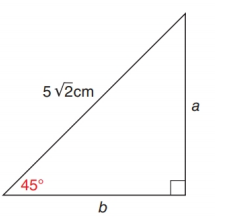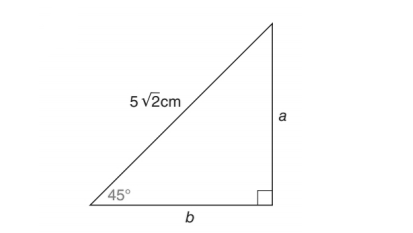Chapter 11.2, Problem 19EElementary Geometry For College St...

7th Edition
Alexander + 2 others
ISBN: 9781337614085

Solutions

Chapter
SectionElementary Geometry For College St...

7th Edition
Alexander + 2 others
ISBN: 9781337614085
Textbook Problem

In Exercise 17 to 22, use either the sine ratio or the cosine ratio to find the length of the indicated sides of the triangle correct to the nearest tenth of a unit.To determine

To find:

The length of the indicated sides of the following triangle,Explanation

Consider the following figure,

General formula for cosine ratio and sin ratio is given below,

From the given figure the value 52cm is the hypotenuse value since it is opposite to right angle.

Then b. is the length of the leg adjacent to 45 and a is the length of leg opposite to 45.

Therefore,

cos45=b52b=52×cos45

Using a scientific calculator in degree mode, follow the following key sequence,

45cos0

Still sussing out bartleby?

Check out a sample textbook solution.

See a sample solution

The Solution to Your Study Problems

Bartleby provides explanations to thousands of textbook problems written by our experts, many with advanced degrees!

Get Started

In Exercises 35-42, find functions f and g such that h = g f. (Note: The answer is not unique.) 40. h(x)=1x24

Applied Calculus for the Managerial, Life, and Social Sciences: A Brief Approach

Find the value of the sum. 32. i=1n(3+2i)2

Single Variable Calculus: Early Transcendentals, Volume I

Differentiate. F(t)=AtBt2+Ct3

Calculus: Early Transcendentals

In Problems 25-34, use the matrices below. Perform the indicated operations.

Mathematical Applications for the Management, Life, and Social Sciences

Find the derivative of the function. F (x) = (1 + x + x2)99

Single Variable Calculus: Early Transcendentals

Finding a Limit In Exercises 47-62, find the limit. limx4x+53x4

Calculus: Early Transcendental Functions (MindTap Course List)

The equation that best describes the curve at the right is:

Study Guide for Stewart's Multivariable Calculus, 8th

True or False: f(a)=lima0f(a+h)f(a)h.

Study Guide for Stewart's Single Variable Calculus: Early Transcendentals, 8th